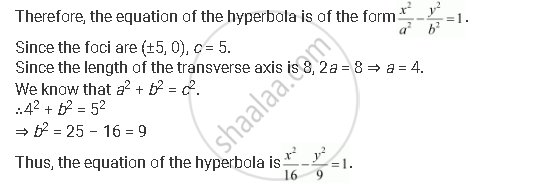CBSE (Arts) Class 11CBSE
Share
Notifications

View all notifications
Books Shortlist
Your shortlist is empty

# Find the Equation of the Hyperbola Satisfying the Give Conditions: Foci (±5, 0), the Transverse Axis is of Length 8. - CBSE (Arts) Class 11 - Mathematics

Login
Create free account

Forgot password?
ConceptHyperbola Standard Equation of Hyperbola

#### Question

Find the equation of the hyperbola satisfying the give conditions: Foci (±5, 0), the transverse axis is of length 8.

#### Solution

Foci (±5, 0), the transverse axis is of length 8.

Here, the foci are on the x-axis.Is there an error in this question or solution?

#### APPEARS IN

Solution Find the Equation of the Hyperbola Satisfying the Give Conditions: Foci (±5, 0), the Transverse Axis is of Length 8. Concept: Hyperbola - Standard Equation of Hyperbola.
S